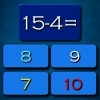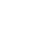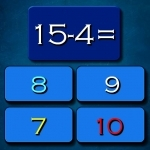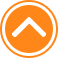×

PlaySubtraction Math Challenge72%Device: 💻 Pc   📱 Mobile
Rating: 72%
🎲Subtraction Math Challenge

Subtraction Math Challenge is fun math game suitable for all ages. I hope you like math and this game is just to test your math skills. Ten seconds to answer each quastion. Have fun playing. Here you can play Subtraction Math Challenge game and watch its walkthrough for free in friv2020.com. Subtraction Math Challenge is an amazing game from our list of Friv 2020 games.

🎮 Instructions

Use Left Mouse button to Play

📂 Categories

puzzle, arcade, mathka, numbers, school, thinking, mathematical, mathematics, igrice, mathematic, math..

👌 Recommended
📌 Tags